CIS 5511. Programming Techniques

Basic Concepts

### 1. Algorithm and data structure

A computational problem is specified as an input/output relationship (or function, mapping), with
1. the scope of the valid input data,
2. the desired input/output relationship.
An algorithm is a procedure of computational steps that transforms an input into an output, where
• the steps are directly executable,
• the procedure is predetermined and has a finite-length description,
• the process will terminate for every valid input.
An algorithm provides a solution for the problem by specifying how to turn the input into the output.

Usually, a problem corresponds to a class containing many instances, and the input/output relation is defined on all the instances. An algorithm for this problem should handle all instances in the class.

For example, "sorting" is a problem where the input is a sequence of items of a certain type, with an order (a transitive relation) defined between any pair of them, and the output should be a sequence of the same items, ordered according to the given relation. A sorting algorithm should specify, step by step, how to turn any valid input into the corresponding output in finite time, then stop there.

An algorithm is correct if for every valid input instance, it halts with the correct output (with respect to the specification of the problem). An algorithm is incorrect if there is a valid input instance for which the algorithm produces an incorrect answer or no answer at all (i.e. does not halt).

For a given problem, if there are multiple candidate algorithms, which one should be used? There are several factors to be considered:

• correctness
• time and space efficiency
• conceptual simplicity
They are usually considered in the above order, though very often a compromise is needed among these factors.

When the (input, output, or intermediate) data of a problem contain multiple items, they are usually organized in a data structure, which represents both the data items and the relation among them.

A data structure can be specified either abstractly, in terms of the operations that can be carried out on it, or concretely, in terms of the storage organization and the algorithms implementing the operations.

In the design and selection of data structures, the analysis of the algorithms involved is a central topic.

Programming means to implement an algorithm in a computer language. A program is language-specific, but an algorithm is language-independent.

### 2. Time efficiency analysis

Traditionally, the focus of algorithm analysis has been on the time efficiency of (correct) algorithms, though space efficiency and the correctness of an algorithm can also be analyzed.

For a given program, the actual time it takes to solve a given problem instance depends on

• the algorithm implemented in the program,
• the given problem instance,
• the software and hardware in which the program is executed.
Since the aim of algorithm analysis is to compare algorithms, the other factors need to be somehow removed from the picture.

The common practice is to define a size for each instance of the problem (which intuitively measures the relative difficulty of the instance), then to represent the number of execution of a certain operation as a function of instance size. Finally, the increasing rate of the function, with respect to the increasing of the instance size, is used as the indicator of the efficiency of the algorithm.

With such a procedure, algorithm analysis becomes a pure mathematical problem, which is well-defined, and the result has universal validity.

Though it is a powerful technique, we need to be aware that many relevant factors have been ignored in this process, and therefore, if for certain reason some of the factors have to be taken into consideration, the traditional algorithm analyzing approach may become improper to use.

Also, some of the decisions made during the formalization process, such as the definition of problem size and the selection of the operation to be counted, are not always obvious or unique, and different decisions may lead to different conclusions.

### 3. Conventions of the pseudocode

The algorithms in the textbook are written in pseudocode, according to the following conventions:
• Indentation indicates block structure, without delimiters.
• The looping and the conditional constructs are similar to those in C/Java/Python/Pascal.
• Double slash ("//") is used for comments.
• Assignment sign is equal sign, "=", and multiple assignment is allowed.
• Double equal sign, "==", is for equality.
• Variables are local by default.
• Array elements are represented as Array[index].
• A field in an object is represented as "object.field", and array length is Array.length.
• Parameters are passed to a procedure by value.
• A return statement transfers control back to the calling procedure.
• The boolean operators "and" and "or" are short circuiting.
For algorithm analysis, we assume the following directly executable instructions:
• arithmetic: add, subtract, multiply, divide, remainder, floor, ceiling;
• data movement: load, store, copy;
• control: conditional and unconditional branch, subroutine call and return.
We also assume that each such instruction takes a constant amount of time. Again, under these assumptions, many relevant factors are ignored.

More complicated blocks, such as loops, can be built from the above instructions.

### 4. Example: sort using an incremental approach

Sorting: the problem and its input/output.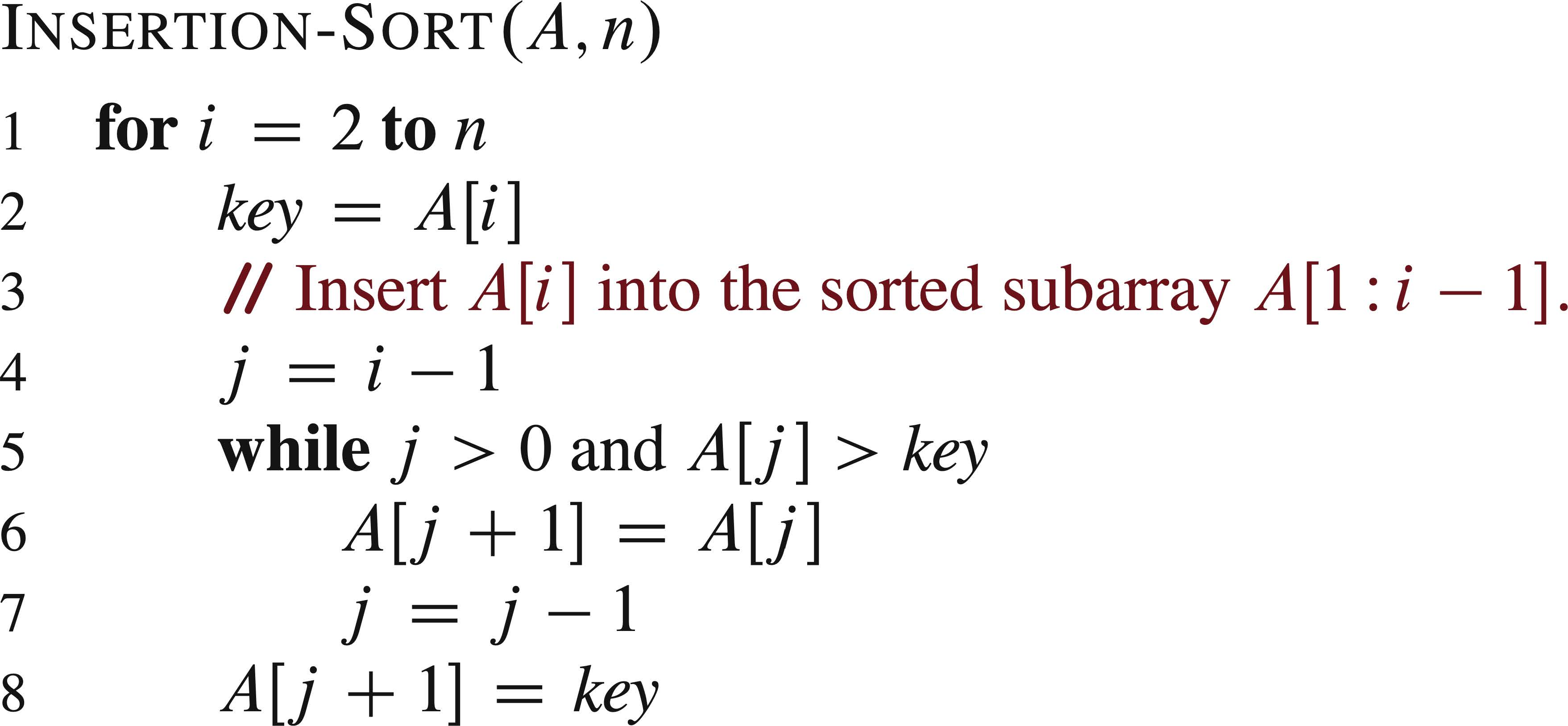The correctness of the algorithm is proven by checking the loop invariant, a proposition about a relation, such as

At the start of each iteration of the for loop of line 1-8, the subarray A[1 .. j − 1] consists of the elements originally in A[1 .. j − 1] but in sorted order.
We must show three things about a loop invariant:
• Initialization: It is true prior to the first iteration of the loop.
• Maintenance: If it is true before an iteration of the loop, it remains true before the next iteration.
• Termination: When the loop terminates, the invariant gives us a useful property that helps show that the algorithm is correct.
These properties hold for the above insertion sort algorithm.

For sorting, it is natural to use the number of keys to be sorted as input size, and it is assumed that a constant amount of time is required to execute each line of our pseudocode (except comments).

Now let us mark the cost and the number of execution times of each line: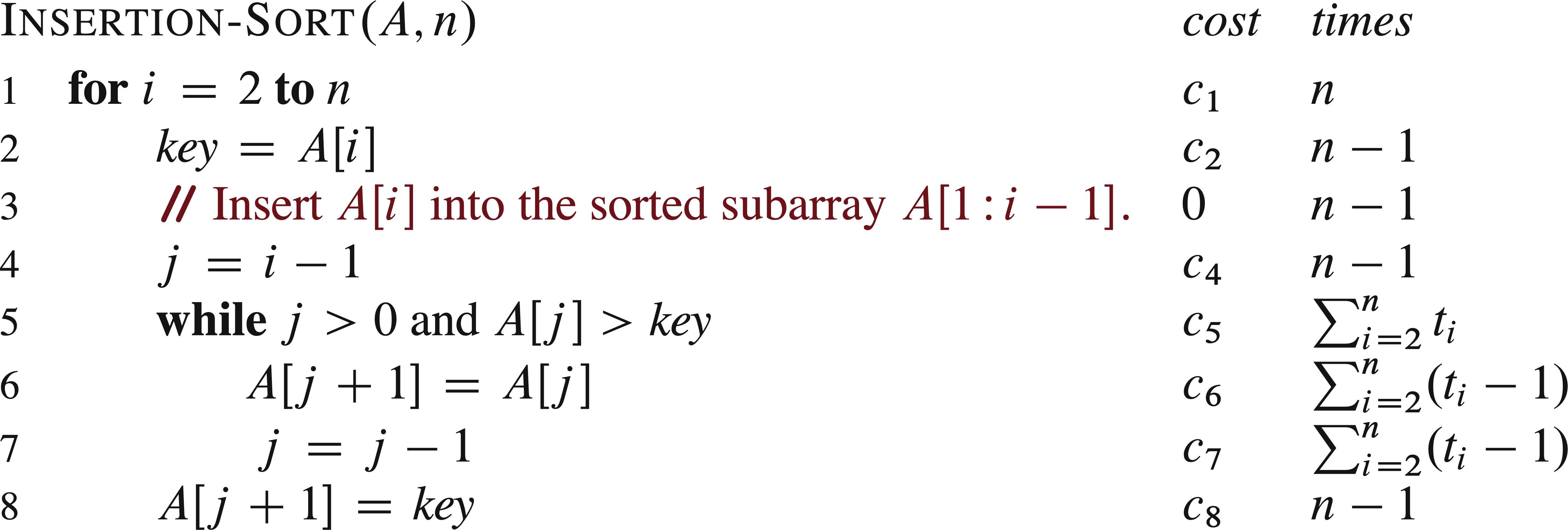in the algorithm, n is length[A]. In line 5-7, tj is the number of times for the while loop test is executed for that number of j.

The running time of the algorithm is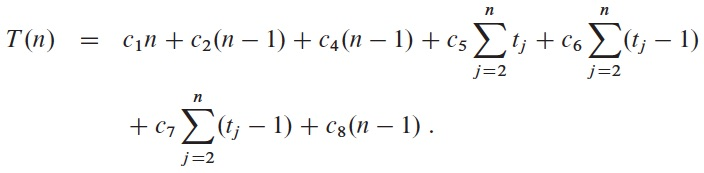For a given n, T(n) depends on the values of tj, which change from instance to instance.

The best case of the algorithm happens when the array is already sorted, so that tj = 1 for j = 2, 3, ..., n, and the function becomes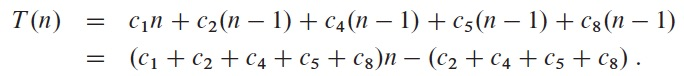which is a linear function of n.

The worst case of the algorithm happens when the array is reverse sorted, so that tj = j for j = 2, 3, ..., n, and the function becomes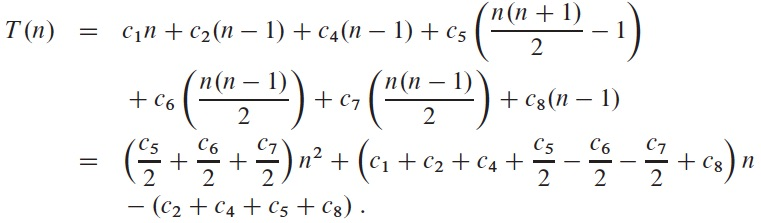which is a quadratic function of n.

Usually, analysis of algorithm is concentrated on the worst case. Though average case is also important, it is harder to analyze.

### 5. Example: sort using a divide-and-conquer approach

The divide-and-conquer approach solves a problem by
1. divide the problem into a number of subproblems,
2. conquer the subproblems (by solving them recursively),
3. combine the solutions to the subproblems into the solution for the original problem.
To apply this approach to sorting, we get "merge sort", which is an algorithm that sorts an array by cutting it into two halves, sorting them recursively, then merge the results.

The merge procedures Merge(A, p, q, r) is given in the following: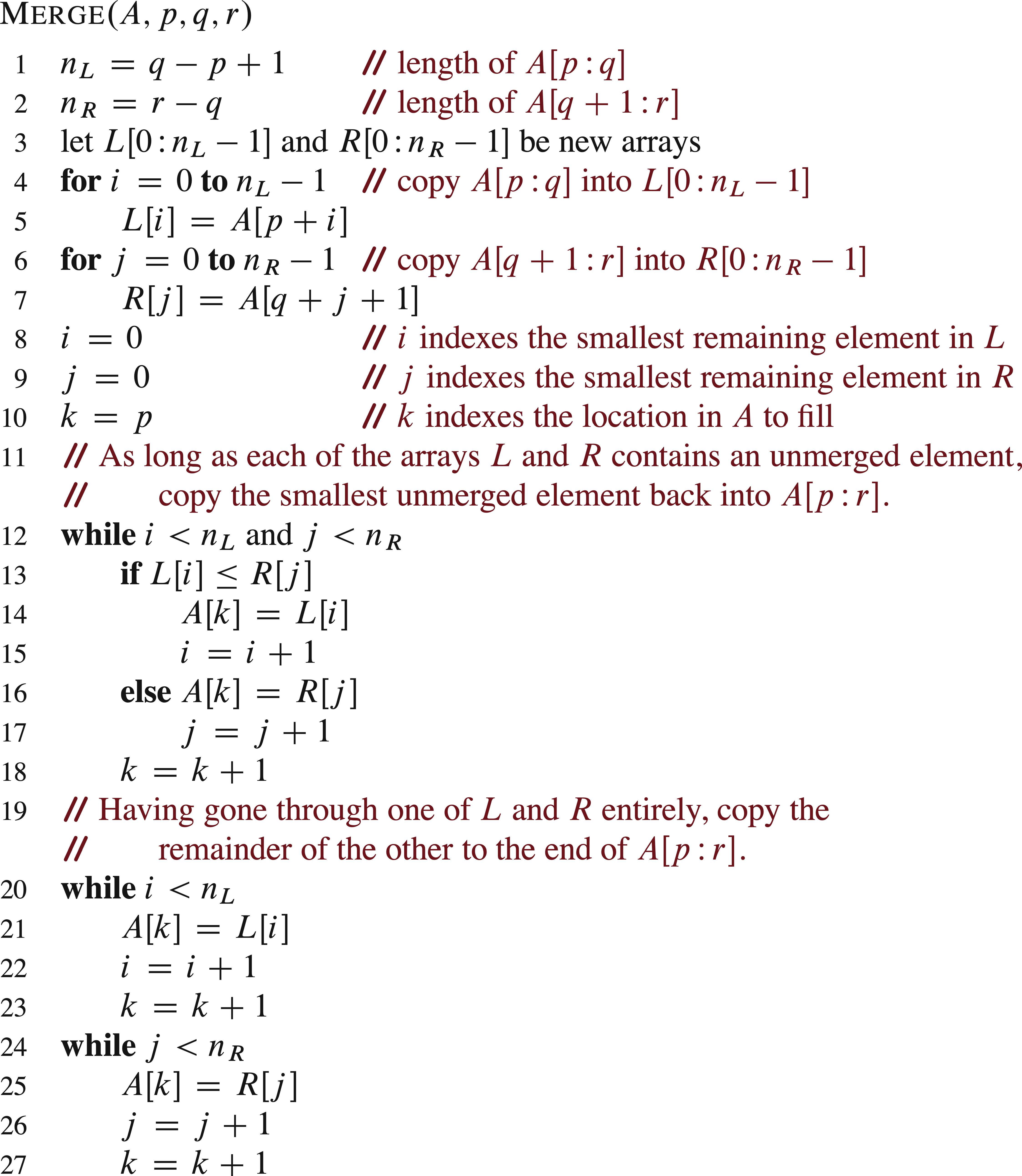It first moves the two (sorted) subarrays A[p...q] and A[q+1...r] into two separate arrays L and R, put two special sentinel values at the end of each of them, then merge the two back into the original array A[p...r]. Its time expense is a linear function of n.

The following is the merge sort algorithm, which recursively calls itself on the two halves of the array, then merge the results together.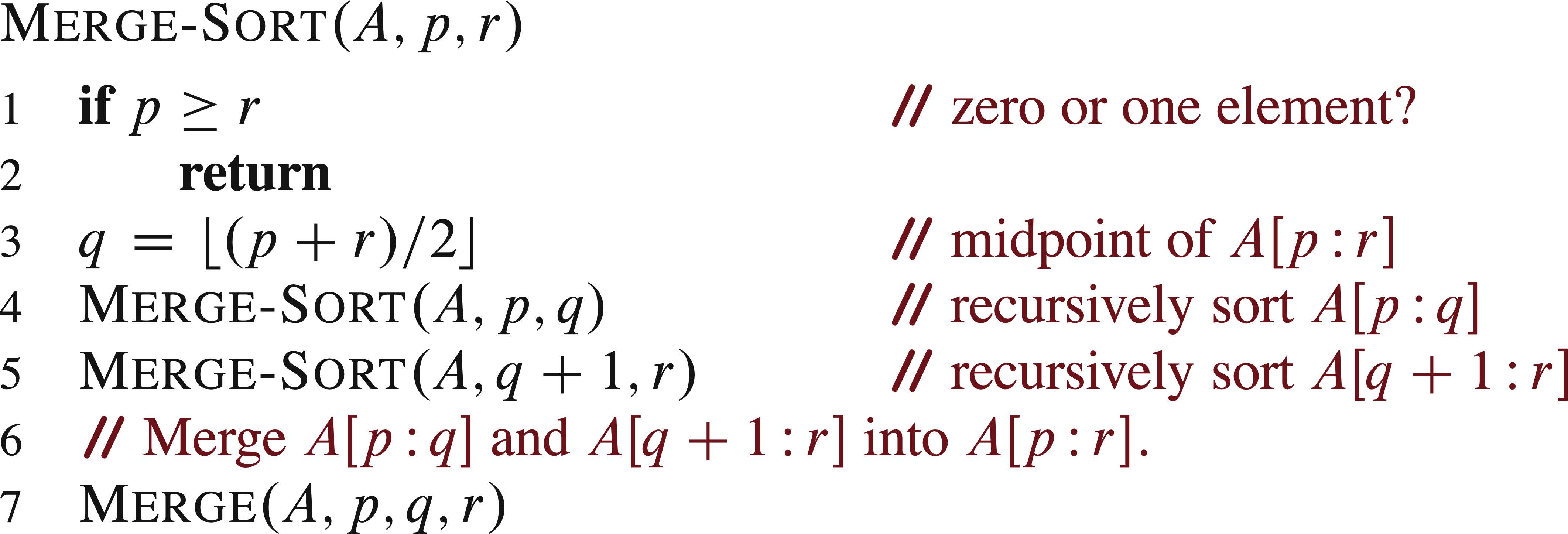The correctness and efficiency of this algorithm can be analyzed similarly.